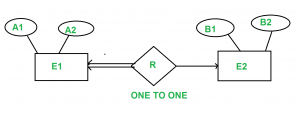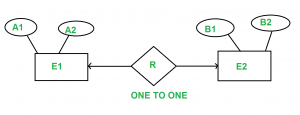# Minimization of ER Diagrams

• Difficulty Level : Medium
• Last Updated : 04 Mar, 2022

Entity-Relationship (ER) Diagram is a diagrammatic representation of data in databases, it shows how data is related.

1) When there are Many to One cardinalities in the ER diagram.
For example, a student can be enrolled only in one course, but a course can be enrolled by many students.For Student(SID, Name), SID is the primary key.  For Course(CID, C_name ), CID is the primary key

```           Student                           Course
(SID   Name)                    ( CID   C_name )
--------------                  -----------------
1      A                        c1      Z
2      B                        c2      Y
3      C                        c3      X
4      D

Enroll
(SID   CID)
----------
1       C1
2       C1
3       c3
4       C2```

Now the question is, what should be the primary key for Enroll? Should it be SID or CID or both combined into one. We can’t have CID as the primary key because a CID can have multiple SIDs. (SID, CID) can distinguish table uniquely, but it is not minimum.  So SID is the primary key for the relation enrollment.

For the above ER diagram, we considered three tables in the database

```Student
Enroll
Course```

But we can combine Student and Enroll table renamed as Student_enroll.

```                 Student_Enroll
( SID   Name   CID )
---------------------
1      A      c1
2      B      c1
3      C      c3
4      D      c2```

Student and enroll tables are merged now.

So require a minimum of two DBMS tables for Student_enroll and Course.

Note: In One to Many relationships we can have a minimum of two tables.

2. When there are Many to Many cardinalities in ER Diagram.
Let us consider the above example with the change that now a student can enroll in more than 1 course.```  Student                            Course
( SID   Name)                    ( CID   C_name )
--------------                  -----------------
1      A                        c1      Z
2      B                        c2      Y
3      C                        c3      X
4      D

Enroll
( SID   CID )
----------
1       C1
1       C2
2       C1
2       C2
3       c3
4       C2```

Now, the same question arises. What is the primary key of Enroll relation? If we carefully analyze, the primary key for Enroll table is ( SID, CID ).

But in this case, we can’t merge Enroll table with any one of the Student and Course. If we try to merge Enroll with any one of the Student and Course it will create redundant data.

Note: Minimum of three tables are required in the Many to Many relationships.

3. One to One Relationship

There are two possibilities
A) If we have One to One relationship and we have total participation at at-least one end.

For example, consider the below ER diagram.A1 and B1 are primary keys of E1 and E2 respectively.

In the above diagram, we have total participation at the E1 end.

Only a single table is required in this case having the primary key of E1 as its primary key.

Since E1 is in total participation, each entry in E1 is related to only one entry in E2, but not all entries in E2 are related to an entry in E1.

The primary key of E1 should be allowed as the primary key of the reduced table since if the primary key of E2 is used, it might have null values for many of its entries in the reduced table.

Note: Only 1 table required.

B) One to One relationship with no total participation.A1 and B1 are primary keys of E1 and E2 respectively.

The primary key of R can be A1 or B1, but we can’t still combine all three tables into one. if we do so, some entries in the combined table may have NULL entries. So the idea of merging all three tables into one is not good.

But we can merge R into E1 or E2.  So a minimum of 2 tables is required.

Below is the Gate Previous Year Question.
https://www.geeksforgeeks.org/gate-gate-cs-2008-question-82/
https://www.geeksforgeeks.org/gate-gate-cs-2008-question-83/